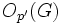# Group of Glauberman type for a prime

The article defines a property of groups, where the definition may be in terms of a particular prime that serves as parameter
View other prime-parametrized group properties | View other group properties

## Definition

### Definition for a general finite group

Suppose$G$ is a finite group and$p$ is a prime number. We say that$G$ is of Glauberman type with respect to$p$ if the ZJ-functor is a characteristic p-functor whose normalizer generates whole group with p'-core. In the discussion below,$Z(J(P))$ denotes the subgroup obtained by applying the ZJ-functor to$P$. The ZJ-functor is defined as the center of the Thompson subgroup$J(P)$, which in turn is defined as the join of abelian subgroups of maximum order.

Explicitly, the following equivalent conditions are satisfied:

1. For one (and hence every)$p$-Sylow subgroup$P$ of$G$,$G = O_{p'}(G)N_G(Z(J(P)))$: Here,$O_{p'}(G)$ denotes the$p'$-core of$G$,
2. For one (and hence every)$p$-Sylow subgroup$P$ of$G$, the image of$Z(J(P))$ in the quotient$G/O_{p'}(G)$ is a normal subgroup of$G/O_{p'}(G)$.
3. For one (and hence every)$p$-Sylow subgroup$Q$ of$K = G/O_{p'}(G)$,$Z(J(Q))$ is a normal subgroup of$K$.
4. For one (and hence every)$p$-Sylow subgroup$Q$ of$K = G/O_{p'}(G)$,$Z(J(Q))$ is a characteristic subgroup of$K$.

### Definition for p'-core-free finite group

Suppose$G$ is a finite group and$p$ is a prime number. Suppose the p'-core$O_{p'}(G)$ is trivial, i.e.,$G$ has no nontrivial normal subgroup of order not divisible by$p$. Then,$G$ is termed a group of Glauberman type for$p$ if it satisfies the following equivalent conditions:

1. For one (and hence every)$p$-Sylow subgroup$P$,$Z(J(P))$ is a normal subgroup of$G$.
2. For one (and hence every)$p$-Sylow subgroup$P$,$Z(J(P))$ is a characteristic subgroup of$G$.

### Equivalence of definitions and its significance

Further information: Equivalence of definitions of group of Glauberman type for a prime

It turns out that, for a finite group$G$ and prime number$p$:$G$ is a group of Glauberman type for$p$$\iff$$G/O_{p'}(G)$ is a group of Glauberman type for$p$

This can be used to provide an alternative definition of group of Glauberman type.

## Examples

### p'-core-free examples

In these example groups,$Z(J(P))$ is normal in$G$ for one (and hence every)$p$-Sylow subgroup$P$ of$G$.

Group Order Prime of interest$p$-Sylow subgroup$Z(J(P))$ Comment
symmetric group:S3 6 3 A3 in S3 A3 in S3 Here,$P = Z(J(P))$ and it itself is normal in$G$.
alternating group:A4 12 2 V4 in A4 V4 in A4 Here,$P = Z(J(P))$ and it itself is normal in$G$.

On the other hand, symmetric group:S4 is not a group of Glauberman type for the prime 2.